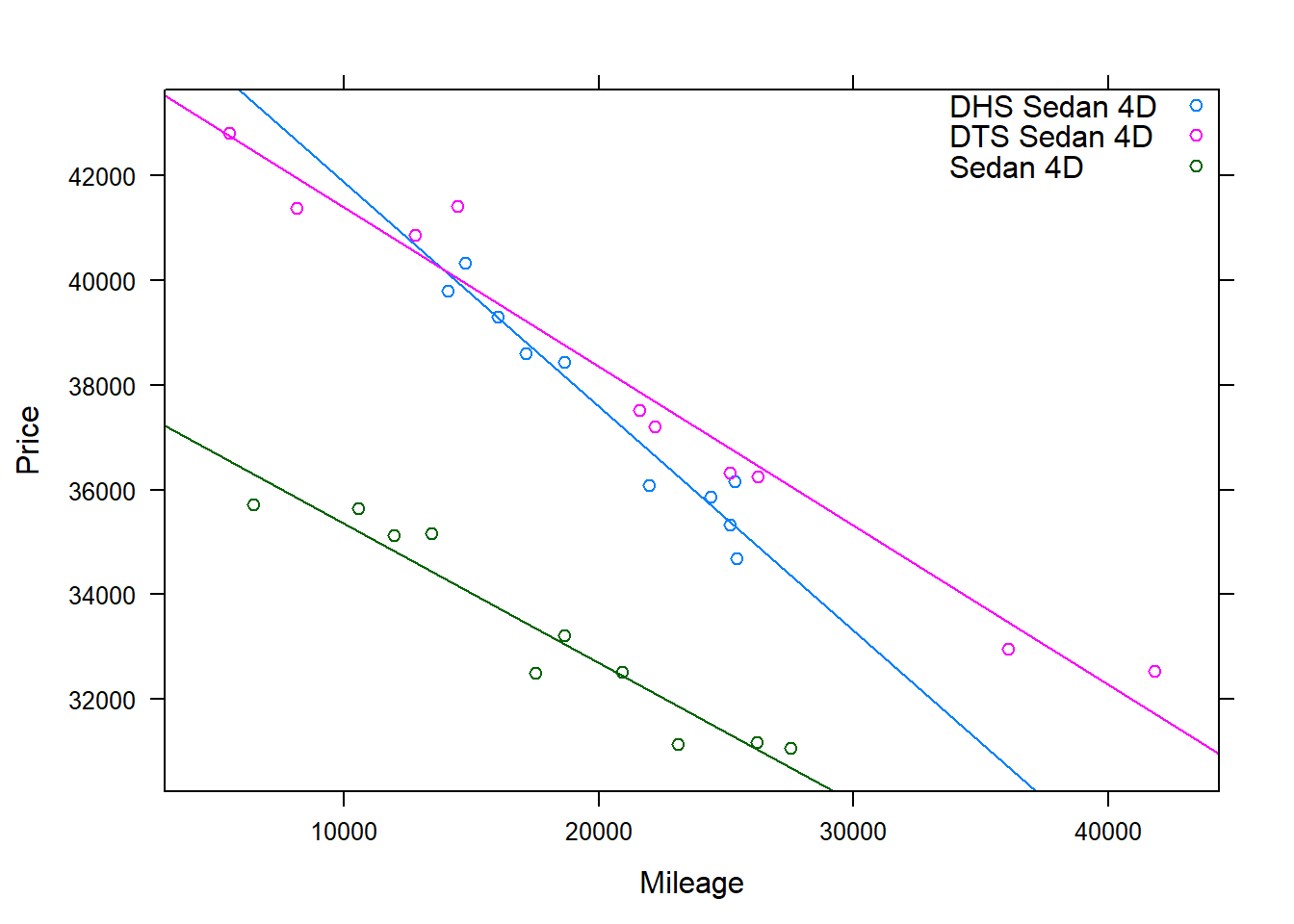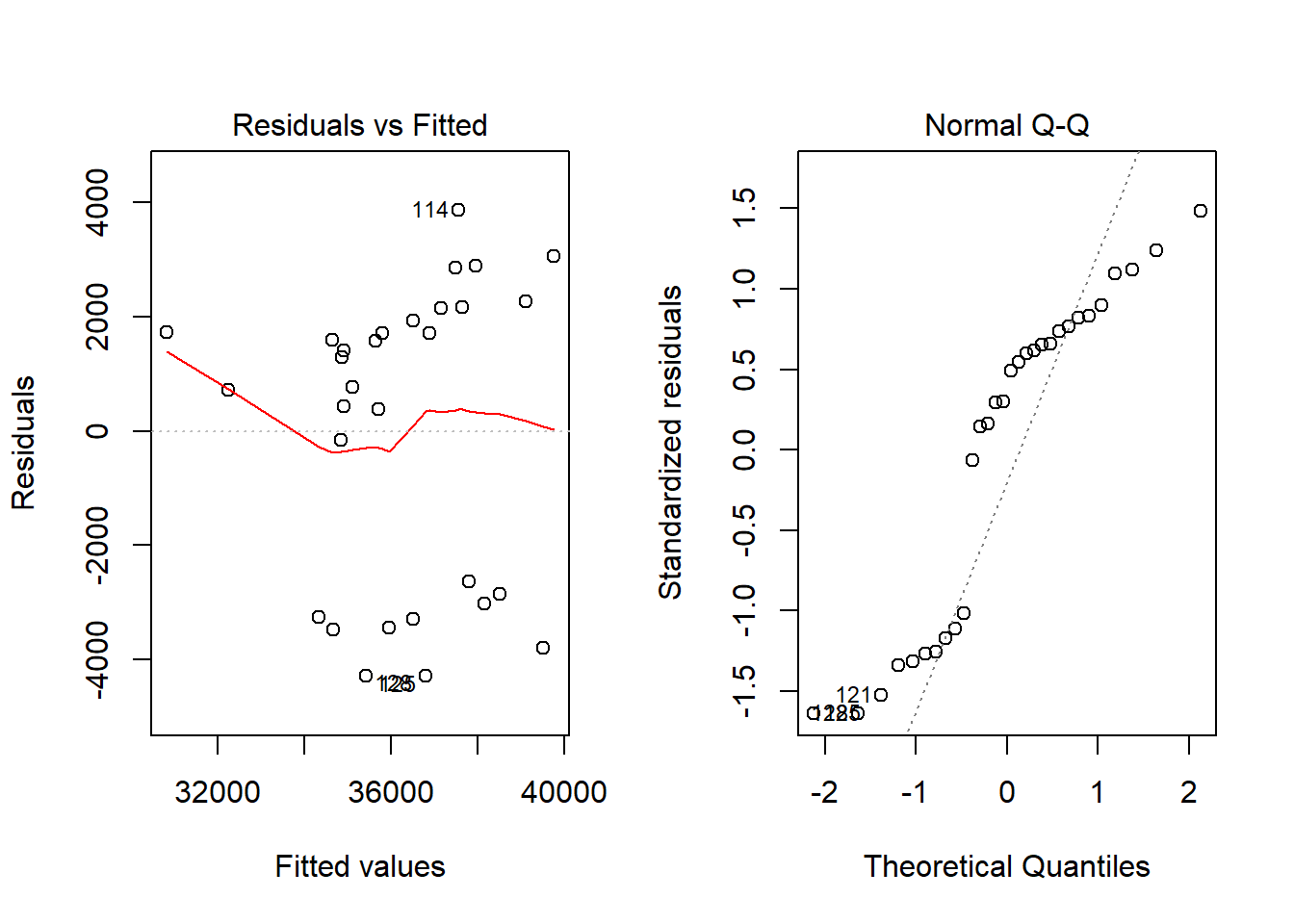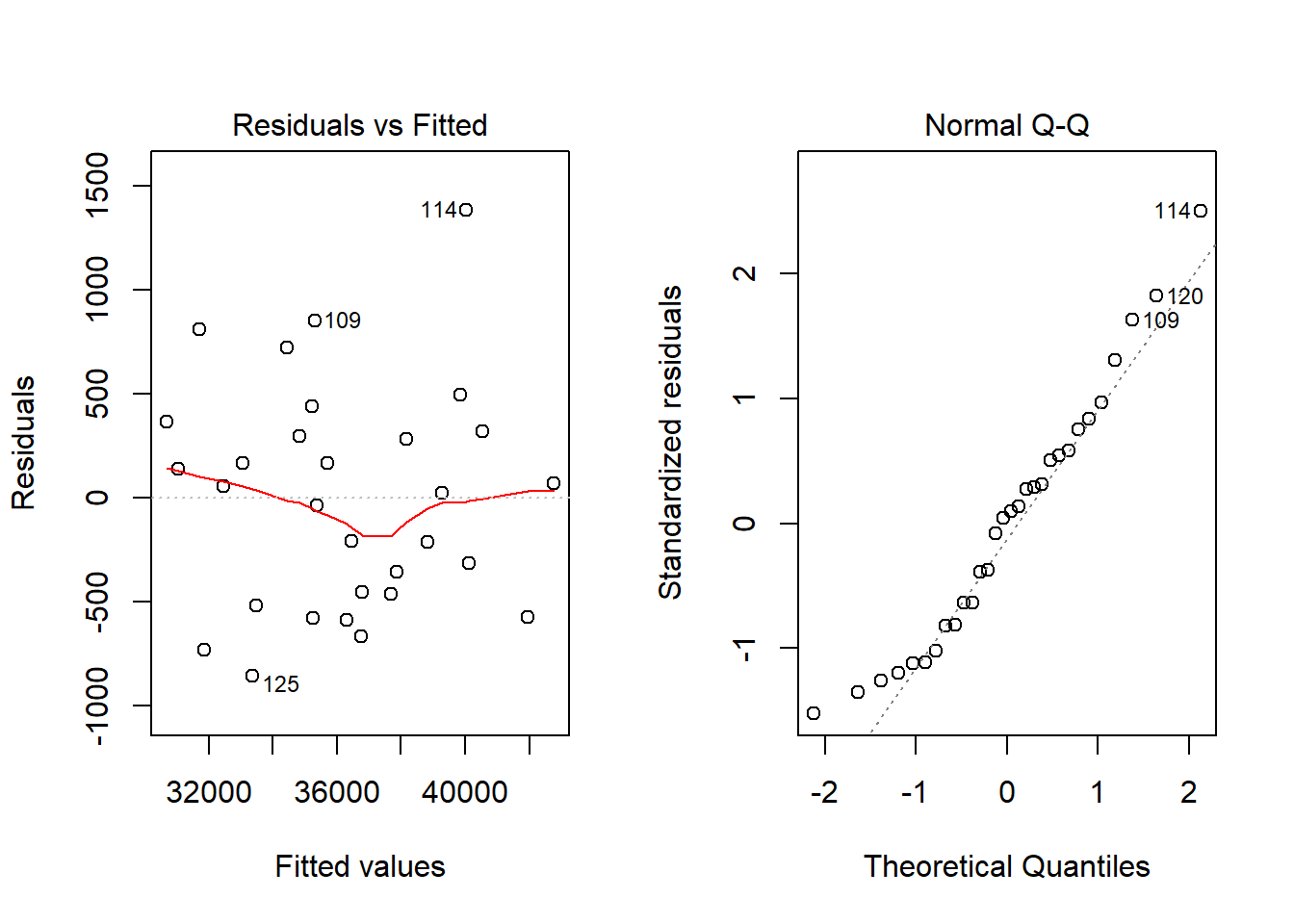## Car Prices for Cadillac Devilles

### Background

The CarPrices dataset containes 804 entires of information on different car makes and models, and different characteristics of such cars, such as price and mileage. The first few lines of data are given below:

knitr::kable(CarPrices[c(1,2,3),])
Price Mileage Make Model Trim Type Cylinder Liter Doors Cruise Sound Leather
17314.10 8221 Buick Century Sedan 4D Sedan 6 3.1 4 1 1 1
17542.04 9135 Buick Century Sedan 4D Sedan 6 3.1 4 1 1 0
16218.85 13196 Buick Century Sedan 4D Sedan 6 3.1 4 1 1 0

In this regression, we saw there were two trends for Devilles. Using a subset of the CarPrices data, we can explore why there are two separate trends. We observe in the dataset that there are three different types of trims for the Deville, the DHS Sedan 4D, the DTS Sedan 4D, and the Sedan 4D. We would like to test whether trim is responsible for variation in the car prices.

### Analysis

We can make a plot of the information to see the different trends in the data. The different colors in the plot represent the three different types of trim.

xyplot( Price ~ Mileage, data = Carsub, groups=Trim, main="", type=c("p","r"), auto.key=list(corner=c(1,1)))It appears that there are different prices of the Devilles based on the trims, but we need to conduct the regressions and view the correlation coefficients to be sure.

First, we should check that the requirements are met. We will need to do this for both the regression with just mileage, and the regression including mileage and trim.

Below are the results for the plots using just price based on mileage:

par(mfrow=c(1,2)); plot(Car2.lm, which=1:2)Whether the requirements are satisfied for regression is very questionable based on these plots. It appears that the relation is not linear and that there is likely unconstant variance. Also, the Q-Q plot shows that normality is also questionable. However, for the purpose of this analysis, we will continue with the test as if the requirements were satisfied.

Now, we want to check the requirements for regression looking at price based on mileage and trim. The plots are shown below:

par(mfrow=c(1,2)); plot(Car.lm, which=1:2)These plots look normal, and the requirements look satisfied. It seems there is linearity, constant variance, and normality. Therefore, we will continue with the analysis.

First, we will conduct a simple linear regression that looks at price by mileage. After conducting the test, we get the following output:

pander(summary(Car2.lm))
Estimate Std. Error t value Pr(>|t|)
Mileage -0.2461 0.0607 -4.055 0.0003624
(Intercept) 41106 1295 31.73 1.706e-23
Fitting linear model: Price ~ Mileage
Observations Residual Std. Error $$R^2$$ Adjusted $$R^2$$
30 2667 0.37 0.3475

The adjusted R squared is 0.3475, which is a weak to moderate positive correlation. Now, we want to conduct a multiple linear regression that looks at price by mileage times trim. After conducting the test, we get the following output.

pander(summary(Car.lm))
Estimate Std. Error t value Pr(>|t|)
Mileage -0.4281 0.04261 -10.05 4.49e-10
TrimDTS Sedan 4D -1731 975.6 -1.774 0.08875
TrimSedan 4D -8132 1035 -7.86 4.308e-08
Mileage:TrimDTS Sedan 4D 0.1246 0.04589 2.716 0.01205
Mileage:TrimSedan 4D 0.1621 0.05122 3.164 0.004185
(Intercept) 46151 885.2 52.13 3.272e-26
Fitting linear model: Price ~ Mileage * Trim
Observations Residual Std. Error $$R^2$$ Adjusted $$R^2$$
30 595.3 0.9731 0.9675

Now, the adjusted R squared is 0.9675. This is a high positive correlation. Because the correlation coefficient was much higher in the second test than the first test, we can conclude that trim adds to the model and is repsonsible for some of the variation in price of Devilles. This supports what we found with our initial plot at the beginning of the analysis.

### Interpretation

From this analysis, we can answer the question: Why were there two different trends of Devilles? It appears that there were two different trends because there were different types of trim for the Deville. We can see from the plot and the analysis, that the regular Sedan 4D was reponsible for the trend with the lower price, and the combination of the DTS Sedan 4D and the DHS Sedan 4D were responsible for the trend with the higher price.

Using the test outputs above, we can also gather more information on the differences between these three types of trim. Each of the types of trim had significant slopes, with the following p-values:

Sedan 4D: 0.004185
DTS Sedan 4D: 0.01205
DHS Sedan 4D: 4.49e-10

This means that each of the types of Deville had a different slope so the price depreciated differently for each type. While the DHS Sedan 4D and the Sedan 4D both had significant Y-intercepts (3.272e-26 and 4.308e-08), the Y-intercept for the DTS Sedan 4D was not significant: 0.08875. This means that with the DHS in the model, the DTS’s Y-intercept wasn’t different, meaning that those two types of Deville cost approximately the same amount when the mileage is 0.

Overall, this analysis shows that for Cadillac Devilles, different types of trim will affect the price of the car. Also, depending on the type of trim, the price of the car will depreciate differently.